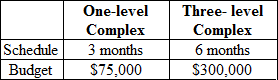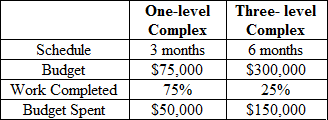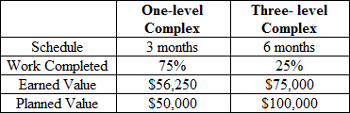Business Courses / Course / Chapter

# Schedule Variance: Definition, Formula & Calculation

Instructor: Toni Bonton

Toni has taught personal finance and has an MBA.

Tracking the status of a project is important in managing any project because the actual schedule may be different from the planned schedule. Schedule variance and how it is applied will be discussed in this lesson.

## The Game Plan

Carlos is a real estate mogul who recently purchased two small apartment complexes. One is a small one-level complex with only 25 units and the other is much larger, with three levels and a total of 475 units. Before Carlos begins leasing to tenants, he wants to do some renovations to give his complexes a more modern look. After several discussions, Carlos and Al, the contractor, finally agree on the following schedule and budget for the renovations:After two months of renovations, Carlos decides to check in on Al to see how the renovations are going. Carlos wants to make sure that the renovations are on track to be completed on schedule and on budget. Al gives Carlos the following report:But these numbers alone are not enough to tell Carlos the status of each project. He needs to calculate the schedule variance. The schedule variance is a status update that indicates how much ahead of, behind, or on schedule a project is based on how much work has been done and how much of the budget has been spent.

## The Formula

To determine the schedule variance, Carlos will need to calculate the difference between the earned value, which is what the project is worth at its current stage of completion, and the planned value, which is what the project is worth based on how much work should be completed. The formula looks like this:

Schedule Variance (SV) = Earned Value (EV) - Planned Value (PV)

Once the planned value is deducted from the earned value, the remaining value reveals whether or not the project is ahead of or behind schedule. A positive schedule variance means the project is ahead of schedule, while a negative schedule variance means that a project is behind schedule. If the schedule variance is zero, the project is on schedule. But this doesn't tell us just how far ahead or behind we are, so let's take it a step further.

Using the schedule variance, Carlos can also calculate to what degree a project is ahead of or behind schedule by dividing the schedule variance by the planned value. The resulting percentage represents just how much the project is ahead of, behind, or on schedule.

Percentage of Schedule Variance (SV%) = (Schedule Variance (SV) / Planned Value (PV)) * 100

Similar to the schedule variance itself, a positive schedule variance percentage reveals to what degree a project is ahead of schedule, while a negative schedule variance percentage reveals to what degree a project is behind schedule. A value of zero indicates that a project is going as planned and on schedule. Let's see these formulas in action.

## Plugging In the Numbers

Carlos is using the information below to calculate the schedule variance and the percentage of the schedule variance.To calculate the schedule variance of the one-level complex, Carlos will deduct the planned value of \$50,000 from the earned value of \$56,250, leaving a schedule variance of \$6,250.

SV = \$56,250 - \$50,000

SV = \$6,250

The positive value lets Carlos know that the renovations on the one-level complex are ahead of schedule. Now Carlos just needs to know how far ahead of schedule Al is with those renovations. Carlos can do this by diving the schedule variance by the planned value.

To unlock this lesson you must be a Study.com Member.

### Register to view this lesson

Are you a student or a teacher?

Back

### Resources created by teachers for teachers

Over 30,000 video lessons & teaching resources‐all in one place.Video lessonsQuizzes & WorksheetsClassroom IntegrationLesson Plans

I would definitely recommend Study.com to my colleagues. It’s like a teacher waved a magic wand and did the work for me. I feel like it’s a lifeline.

Jennifer B.
TeacherCreate an account to start this course today
Used by over 30 million students worldwide